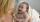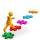# Grandfather and grandmother

The old mother is 5 years younger than the old father. Together they are 153 years old. How many years has each of them?

Result

a =  74
b =  79

#### Solution:

a=b-5
a+b=153

a-b = -5
a+b = 153

a = 74
b = 79

Calculated by our linear equations calculator.

Leave us a comment of this math problem and its solution (i.e. if it is still somewhat unclear...):Be the first to comment!#### Following knowledge from mathematics are needed to solve this word math problem:

Do you have a system of equations and looking for calculator system of linear equations?

## Next similar math problems:

1. Mother and daughter 2The mother is 40 years older than her daughter. How old is the mother if her age is eight thirds age of daughter?
2. Father and sonWhen I was 11, my father was 35 years old. Today, my father has three times more years than me. How old is she?
3. Greg and BillGreg is 18 years old. He is 6 less than 4 times Bill's age. How old is Bill?
4. Ages 2A man's age is 4 times his son's age. After 5 years he will be just twice his son's age, find their ages.
5. Two trainsThere were 159 freight wagons on the railway station creating 2 trains. One had 15 more wagons than the other. How many wagons did each train have?
6. Journey to schoolOn the way to school I went steady step. In the first half of the journey, I counted every second step, in the second half every third. How many steps I do when I went to school if I counted double step are 250 more than three steps?
7. Boys and girlsThere are 48 children in the sports club, boys are 10 more than girls. How many girls go to the club?
8. Waiting roomIn the waiting room are people and flies. Together they have 15 heads and 50 legs (fly has 6 legs). How many people and flies are in the waiting room?
9. WeightsMarry and John together weighing 49 kg. Their weights are in ratio 1:6. Determine their weights.
10. Linear systemSolve this linear system (two linear equations with two unknowns): x+y =36 19x+22y=720
11. Jane plantsJane plants flowers in the garden. If she planted 12 every hour instead of 9 flowers, she would finish with the job an hour earlier. How many flowers does she plant?
12. Two numbersFind two numbers whose difference and ratio is 2.
13. HostelStudents are accommodated in 22 rooms. Rooms were 4 and 6 bed. How many rooms in which type occupied 106 children there?
14. Simple equationsSolve system of equations: 5x+3y=5 5x+7y=25
15. Masons1 mason casts 30.8 meters square in 8 hours. How long casts 4 masons 178 meters square?
16. Spain vs USASpain lost to the US by 4 goals. In the match total fell 10 goals. How many goals gave the Spain and how the United States?
17. ClassIn a class are 32 pupils. Of these are 8 boys. What percentage of girls are in the class?